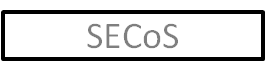# Simple Evolving Connectionist Systems (SECoS)## SECoS Structure

The Simple Evolving Connectionist System (SECoS) was created as a minimalist implementation of the ECoS algorithm, that is, it is an architecture that has the minimum number of neuron layers necessary to learn data. Alternatively, SECoS can be viewed as a minimalist EFuNN, with the fuzzification and defuzzification components being removed. Lacking the fuzzification and defuzzification mechanisms of EFuNN, the SECoS model was created for several reasons. Firstly, they are intended as a simpler alternative to EFuNN. Since they lack the fuzzification and defuzzification structures of EFuNN, SECoS are much simpler to implement. Having fewer connection matrices and a smaller number of neurons, there is much less processing involved in simulating a SECoS network. They are also much easier to understand and analyse: while EFuNN expands the dimensionality of the input and output spaces with its fuzzy logic elements, SECoS deals with the input and output space "as is". Therefore, rather than dealing with a fuzzy problem space, SECoS deals with the problem space directly. Each neuron that is added to the network during training represents a point in the problem space, rather than a point in the expanded fuzzy problem space. Secondly, for some situations, fuzzified inputs are not only unnecessary but harmful to performance, as they lead to an increase in the dimensionality of the input space and hence an increase in the number of evolving layer neurons. Binary data sets are particularly vulnerable to this, as fuzzification does nothing but increase the dimensionality of the input data. By removing the fuzzification and defuzzification capabilities, the adaptation advantages of EFuNN are retained while eliminating the disadvantages of fuzzification, specifically by eliminating the need to select the number and parameters of the input and output membership functions. For most applications, SECoS are able to model the training data with fewer neurons in the evolving layer than an equivalent EFuNN.

A SECoS network consists of three layers of neurons: The input layer, with linear transfer functions; The evolving layer; And an output layer with a simple saturated linear activation function. The distance measure used in the evolving layer is the normalised Manhattan distance, as shown in the following equation:

${D}_{n}=\frac{\sum _{i=1}^{c}\mid {I}_{i}-{w}_{i,n}\mid }{\sum _{i=1}^{c}\mid {I}_{i}+{W}_{i,n}\mid }$

Where:

$c$ is the number of inputs neurons in the SECoS

$I$ is the input vector

$W$ is the input to evolving layer weight matrix

There are two layers of connections in the SECoS model. The first connects the input neuron layer to the evolving layer. The weight values here represent the coordinates of the point in input space each evolving layer neuron represents. The second layer of connections connects the evolving layer to the output neuron layer. The weights in this layer represent the output values associated with the input examples.

The general ECoS learning algorithm is used to train SECoS. In SECoS the input vector is the actual crisp input vector, while the desired outputs vector is the crisp target output vector.

## Fuzzy Rule Extraction

Although SECoS do not have fuzzy logic elements within their structure, it is possible to extract fuzzy rules from them using external MF. The idea behind this approach was that there is no practical difference between the fuzzy exemplars in an EFuNN, where those exemplars have been fuzzified by the EFuNN internal MF, and using external MF to fuzzify the crisp exemplars stored within a SECoS. The algorithm for extracting Zadeh-Mamdani fuzzy rules from trained SECoS networks is as follows:

for each evolving layer neuron $h$ do

Create a new rule $r$

for each input neuron $i$ do

Find the MF $u$ associated with $i$ that activates the most strongly for ${W}_{i,h}$

Add an antecedent to $r$ of the form "$i$ is $u$ $u\left({W}_{i,h}\right)$", where $u\left({W}_{i,h}\right)$ is the confidence factor for the antecedent

end for

for each output neuron $o$ do

Find the MF $u$ associated with $o$ that activates the most strongly for ${W}_{h,o}$

Add a consequent to $r$ of the form "$o$ is $u$ $u\left({W}_{h,o}\right)$", where $u\left({W}_{h,o}\right)$ is the confidence factor for the consequent

end for

end for

Functionally, this algorithm is equivalent to the EFuNN fuzzy rule extraction algorithm. The EFuNN rule extraction algorithm chooses antecedent MF based on the highest magnitude weights from the condition to rule neurons, which are really crisp exemplar values that have been fuzzified by the EFuNNs internal MF. The SECoS fuzzy rule extraction algorithm chooses antecedent MF based on the fuzzified values of the weights, which while representing crisp exemplars, are fuzzified using the provided external MF.

The advantage of this algorithm is that, since the membership functions are not an integral part of the network, the number of MF, their type and their parameters can all be optimised before the rule extraction process is carried out. If the rules extracted with a particular set of MF are not optimal, then the MF can be changed and fresh rules generated, without altering the SECoS.

Maintained by Michael J. Watts